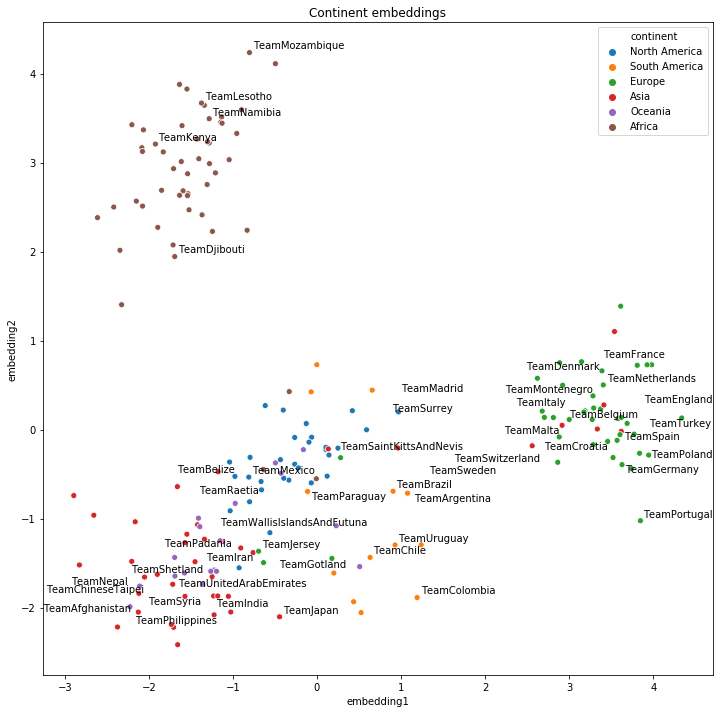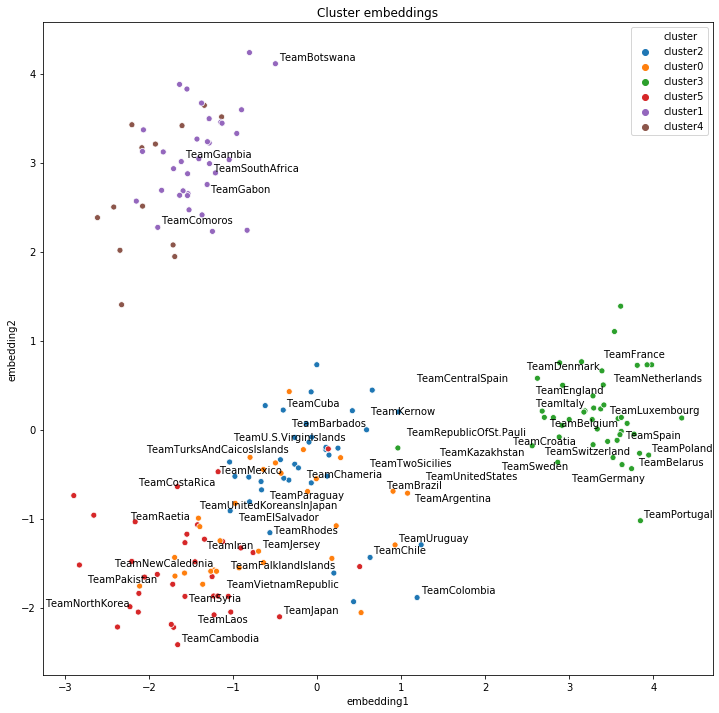# Examples¶

These examples show how to get started with AmpliGraph APIs, and cover a range of useful tasks. Note that additional tutorials are also available.

## Train and evaluate an embedding model¶

import numpy as np
from ampligraph.latent_features import ComplEx
from ampligraph.evaluation import evaluate_performance, mrr_score, hits_at_n_score

def main():

# Initialize a ComplEx neural embedding model with pairwise loss function:
# The model will be trained for 300 epochs.
model = ComplEx(batches_count=10, seed=0, epochs=20, k=150, eta=10,
# Use adam optimizer with learning rate 1e-3
# Use pairwise loss with margin 0.5
loss='pairwise', loss_params={'margin':0.5},
# Use L2 regularizer with regularizer weight 1e-5
regularizer='LP', regularizer_params={'p':2, 'lambda':1e-5},
# Enable stdout messages (set to false if you don't want to display)
verbose=True)

# For evaluation, we can use a filter which would be used to filter out
# positives statements created by the corruption procedure.
# Here we define the filter set by concatenating all the positives
filter = np.concatenate((X['train'], X['valid'], X['test']))

# Fit the model on training and validation set
model.fit(X['train'],
early_stopping = True,
early_stopping_params = \
{
'x_valid': X['valid'],       # validation set
'criteria':'hits10',         # Uses hits10 criteria for early stopping
'burn_in': 100,              # early stopping kicks in after 100 epochs
'check_interval':20,         # validates every 20th epoch
'stop_interval':5,           # stops if 5 successive validation checks are bad.
'x_filter': filter,          # Use filter for filtering out positives
'corruption_entities':'all', # corrupt using all entities
'corrupt_side':'s+o'         # corrupt subject and object (but not at once)
}
)

# Run the evaluation procedure on the test set (with filtering).
# To disable filtering: filter_triples=None
# Usually, we corrupt subject and object sides separately and compute ranks
ranks = evaluate_performance(X['test'],
model=model,
filter_triples=filter,
use_default_protocol=True, # corrupt subj and obj separately while evaluating
verbose=True)

# compute and print metrics:
mrr = mrr_score(ranks)
hits_10 = hits_at_n_score(ranks, n=10)
print("MRR: %f, [email protected]: %f" % (mrr, hits_10))
# Output: MRR: 0.886406, [email protected]: 0.935000

if __name__ == "__main__":
main()


## Model selection¶

from ampligraph.datasets import load_wn18
from ampligraph.latent_features import ComplEx
from ampligraph.evaluation import select_best_model_ranking

def main():

model_class = ComplEx

# Use the template given below for doing grid search.
param_grid = {
"batches_count": ,
"seed": 0,
"epochs": ,
"k": [100, 50],
"eta": [5,10],
# We take care of mapping the params to corresponding classes
"loss_params": {
#margin corresponding to both pairwise and adverserial loss
"margin": [0.5, 20],
"alpha": [0.5]
},
"embedding_model_params": {
# generate corruption using all entities during training
"negative_corruption_entities":"all"
},
"regularizer": [None, "LP"],
"regularizer_params": {
"p": ,
"lambda": [1e-4, 1e-5]
},
"optimizer_params":{
"lr": [0.01, 0.0001]
},
"verbose": True
}

# Train the model on all possibile combinations of hyperparameters.
# Models are validated on the validation set.
# It returnes a model re-trained on training and validation sets.
best_model, best_params, best_mrr_train, \
ranks_test, mrr_test = select_best_model_ranking(model_class, # Class handle of the model to be used
# Dataset
X_dict['train'],
X_dict['valid'],
X_dict['test'],
# Parameter grid
param_grid,
# Use filtered set for eval
use_filter=True,
# corrupt subject and objects separately during eval
use_default_protocol=True,
# Log all the model hyperparams and evaluation stats
verbose=True)
print(type(best_model).__name__, best_params, best_mrr_train, mrr_test)

if __name__ == "__main__":
main()


## Get the embeddings¶

import numpy as np
from ampligraph.latent_features import ComplEx

model = ComplEx(batches_count=1, seed=555, epochs=20, k=10)
X = np.array([['a', 'y', 'b'],
['b', 'y', 'a'],
['a', 'y', 'c'],
['c', 'y', 'a'],
['a', 'y', 'd'],
['c', 'y', 'd'],
['b', 'y', 'c'],
['f', 'y', 'e']])
model.fit(X)
model.get_embeddings(['f','e'], embedding_type='entity')


## Save and restore a model¶

import numpy as np
from ampligraph.latent_features import ComplEx
from ampligraph.utils import save_model, restore_model

model = ComplEx(batches_count=2, seed=555, epochs=20, k=10)

X = np.array([['a', 'y', 'b'],
['b', 'y', 'a'],
['a', 'y', 'c'],
['c', 'y', 'a'],
['a', 'y', 'd'],
['c', 'y', 'd'],
['b', 'y', 'c'],
['f', 'y', 'e']])

model.fit(X)

#  Use the trained model to predict
y_pred_before = model.predict(np.array([['f', 'y', 'e'], ['b', 'y', 'd']]))
print(y_pred_before)
#[-0.29721245, 0.07865551]

# Save the model
example_name = "helloworld.pkl"
save_model(model, model_name_path = example_name)

# Restore the model
restored_model = restore_model(model_name_path = example_name)

# Use the restored model to predict
y_pred_after = restored_model.predict(np.array([['f', 'y', 'e'], ['b', 'y', 'd']]))
print(y_pred_after)
# [-0.29721245, 0.07865551]


## Split dataset into train/test or train/valid/test¶

import numpy as np
from ampligraph.evaluation import train_test_split_no_unseen

'''
Assume we have a knowledge graph stored in my_folder/my_graph.csv,
and that the content of such file is:

a,y,b
f,y,e
b,y,a
a,y,c
c,y,a
a,y,d
c,y,d
b,y,c
f,y,e
'''

# Load the graph in memory

# To split the graph in train and test sets:
# (In this toy example the test set will include only two triples)
X_train, X_test = train_test_split_no_unseen(X, test_size=2)

print(X_train)

'''
X_train:[['a' 'y' 'b']
['f' 'y' 'e']
['b' 'y' 'a']
['c' 'y' 'a']
['c' 'y' 'd']
['b' 'y' 'c']
['f' 'y' 'e']]
'''

print(X_test)

'''
X_test: [['a' 'y' 'c']
['a' 'y' 'd']]
'''

# To split the graph in train, validation, and test the method must be called twice:
X_train_valid, X_test = train_test_split_no_unseen(X, test_size=2)
X_train, X_valid = train_test_split_no_unseen(X_train_valid, test_size=2)

print(X_train)
'''
X_train:  [['a' 'y' 'b']
['b' 'y' 'a']
['c' 'y' 'd']
['b' 'y' 'c']
['f' 'y' 'e']]
'''

print(X_valid)
'''
X_valid:  [['f' 'y' 'e']
['c' 'y' 'a']]
'''

print(X_test)
'''
X_test:  [['a' 'y' 'c']
['a' 'y' 'd']]
'''


## Clustering and projectings embeddings into 2D space¶

### Embedding training¶

import numpy as np
import pandas as pd
import requests

from ampligraph.latent_features import ComplEx
from ampligraph.evaluation import evaluate_performance
from ampligraph.evaluation import mr_score, mrr_score, hits_at_n_score
from ampligraph.evaluation import train_test_split_no_unseen

# International football matches triples
url = 'https://ampligraph.s3-eu-west-1.amazonaws.com/datasets/football.csv'
open('football.csv', 'wb').write(requests.get(url).content)
X = load_from_csv('.', 'football.csv', sep=',')[:, 1:]

# Train test split
X_train, X_test = train_test_split_no_unseen(X, test_size=10000)

# ComplEx model
model = ComplEx(batches_count=50,
epochs=300,
k=100,
eta=20,
optimizer_params={'lr':1e-4},
loss='multiclass_nll',
regularizer='LP',
regularizer_params={'p':3, 'lambda':1e-5},
seed=0,
verbose=True)

model.fit(X_train)


### Embedding evaluation¶

filter_triples = np.concatenate((X_train, X_test))
ranks = evaluate_performance(X_test,
model=model,
filter_triples=filter_triples,
use_default_protocol=True,
verbose=True)

mr = mr_score(ranks)
mrr = mrr_score(ranks)

print("MRR: %.2f" % (mrr))
print("MR: %.2f" % (mr))

hits_10 = hits_at_n_score(ranks, n=10)
print("[email protected]: %.2f" % (hits_10))
hits_3 = hits_at_n_score(ranks, n=3)
print("[email protected]: %.2f" % (hits_3))
hits_1 = hits_at_n_score(ranks, n=1)
print("[email protected]: %.2f" % (hits_1))
'''
MRR: 0.25
MR: 4927.33
[email protected]: 0.35
[email protected]: 0.28
[email protected]: 0.19
'''


### Clustering and 2D projections¶

Please install lib adjustText first with pip install adjustText. For incf.countryutils, do the following steps:

git clone https://github.com/wyldebeast-wunderliebe/incf.countryutils.git
cd incf.countryutils
pip install .


incf.countryutils is used to map countries to the corresponding continents.

import re
from sklearn.decomposition import PCA
from sklearn.cluster import KMeans
import matplotlib.pyplot as plt
import seaborn as sns
from incf.countryutils import transformations
from ampligraph.discovery import find_clusters

# Get the teams entities and their corresponding embeddings
triples_df = pd.DataFrame(X, columns=['s', 'p', 'o'])
teams = triples_df.s[triples_df.s.str.startswith('Team')].unique()
team_embeddings = dict(zip(teams, model.get_embeddings(teams)))
team_embeddings_array = np.array([i for i in team_embeddings.values()])

# Project embeddings into 2D space via PCA
embeddings_2d = PCA(n_components=2).fit_transform(team_embeddings_array)

# Cluster embeddings (on the original space)
clustering_algorithm = KMeans(n_clusters=6, n_init=100, max_iter=500, random_state=0)
clusters = find_clusters(teams, model, clustering_algorithm, mode='entity')

# This function maps country to continent
def cn_to_ctn(country):
try:
original_name = ' '.join(re.findall('[A-Z][^A-Z]*', country[4:]))
return transformations.cn_to_ctn(original_name)
except KeyError:
return "unk"

plot_df = pd.DataFrame({"teams": teams,
"embedding1": embeddings_2d[:, 0],
"embedding2": embeddings_2d[:, 1],
"continent": pd.Series(teams).apply(cn_to_ctn),
"cluster": "cluster" + pd.Series(clusters).astype(str)})

# Top 20 teams in 2019 according to FIFA rankings
top20teams = ["TeamBelgium", "TeamFrance", "TeamBrazil", "TeamEngland", "TeamPortugal",
"TeamCroatia", "TeamSpain", "TeamUruguay", "TeamSwitzerland", "TeamDenmark",
"TeamArgentina", "TeamGermany", "TeamColombia", "TeamItaly", "TeamNetherlands",
"TeamChile", "TeamSweden", "TeamMexico", "TeamPoland", "TeamIran"]

np.random.seed(0)

# Plot 2D embeddings with country labels
def plot_clusters(hue):
plt.figure(figsize=(12, 12))
plt.title("{} embeddings".format(hue).capitalize())
ax = sns.scatterplot(data=plot_df[plot_df.continent!="unk"],
x="embedding1", y="embedding2", hue=hue)
texts = []
for i, point in plot_df.iterrows():
if point["teams"] in top20teams or np.random.random() < 0.1:
texts.append(plt.text(point['embedding1']+0.02,
point['embedding2']+0.01,
str(point["teams"])))

plot_clusters("continent")